# 流量控制 - 从原理到实现

• 2021 年 12 月 15 日
• 本文字数：6465 字

阅读完需：约 21 分钟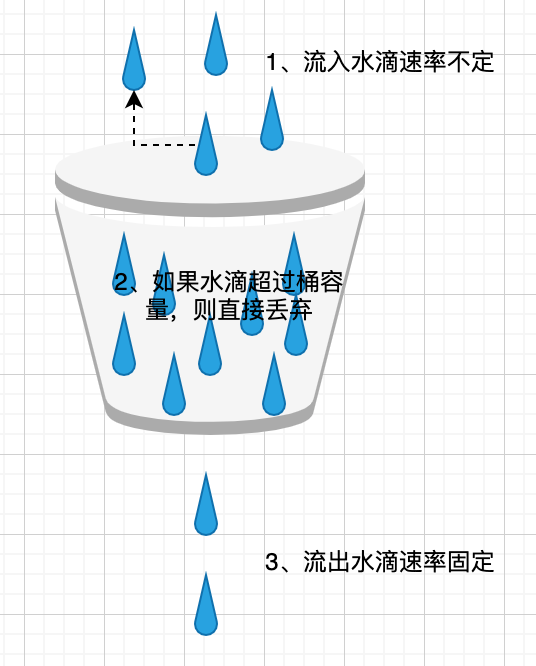## 概念

• 每秒总共传输量，在 1 秒内只要不超过 100m bits，能传多快就多块

• 按照位来平均，每传 1bit 需要花费 0.01ns，因此没传完 1bits 后必须得到 0.01ns 后才能继续传

• 只要 60 秒内部超过 60*100Mb 就行

• ....

• 如何定义流量的计算方式？是选择 1s、10s 还是 60s？

• 如果流量超了之后，该怎么做？是直接返回空值还是一个默认值？

## 常用方式

• 计数器

• 滑动窗口

• 漏桶

• 令牌桶

### 计数器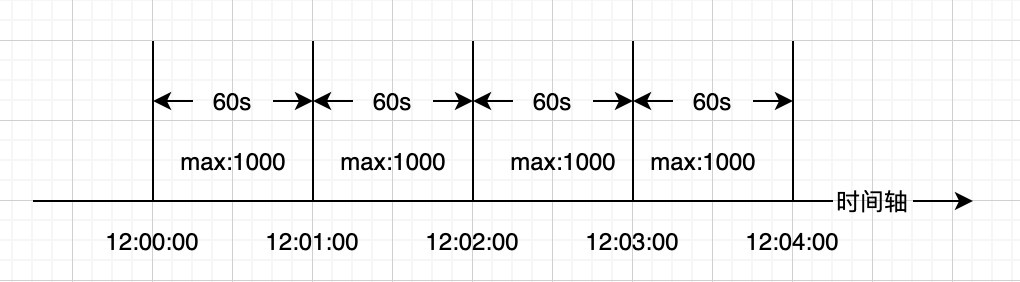#### 原理

• 设置单位时间 T（如 10s）内的最大访问量 req_max，在单位时间 T 内维护计数器 count；

• 当请求到达时，判断时间是否进入下一个单位时间；

• 如果是，则重置计数器为 0；

• 如果不是，计数器 count,并判断计数器 count 是否超过最大访问量 req_max，如超过，则拒绝访问。

#### 实现

class CounterController { public:  CounterController(int max, int duration) {    max_ = max;    duration_ = duration;    last_update_time_ = time(nullptr);  }  bool IsValid() {     uint64_t now = time(nullptr);     if (now < last_update_time_ + duration_) {        ++req_num_;        return max_ > req_num_;     } else {       last_update_time_ = now;       req_num_ = 1;       return max_ > req_num_;     }  } private:  int max_;  int duration_;  int last_update_time_;  int req_num_ = 0;};

• max_ 代表时间片内最多处理的请求个数

• duration_ 代码时间片，单位为秒

• last_update_time_ 上一次更新计数器的时间

• req_num_ 当前时间片内的处理的请求数

#### 特点

• 优点：实现简单，容易理解

• 缺点：

• 一段时间内（不超过时间窗口）系统服务不可用。以图一为例，时间片为 60s，在时间片内的第一秒内，处理请求量就达到了上限，那么在后面的 59s 内，所有的请求都将被拒绝

• 在时间片切换时刻，可能会产生两倍于上限的请求。仍然以图一为例，时间片为 60s，在时间片内的前 59s 都无请求过来，在第 60s 的时候来了 1000 个请求，然后时间片切换，在新的时间片内的第一秒内，来了 1000 个请求，也就是说在 2 秒内(上一个时间片的最后一秒 + 当前时间片的第一秒)来了 2000 个请求，这个时候明显超过我们的时间片内的上限值，可能导致系统崩溃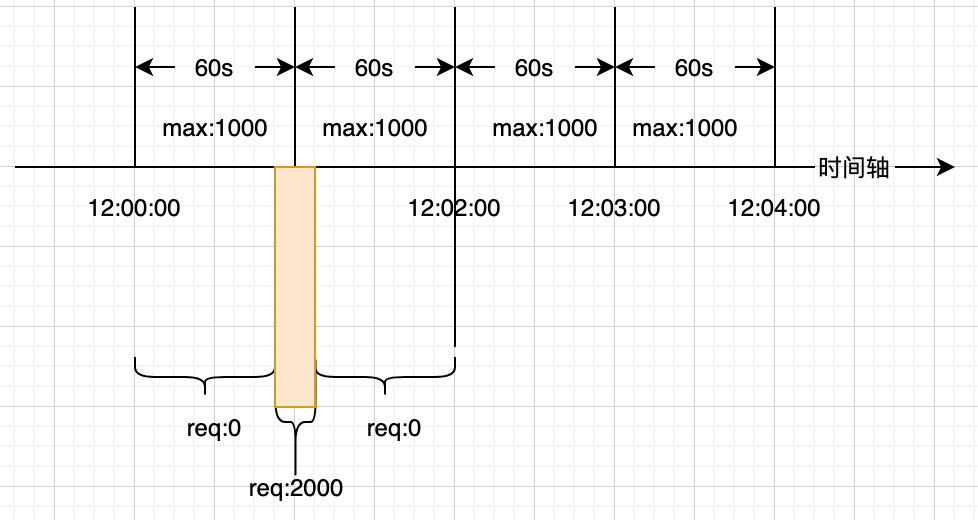### 滑动窗口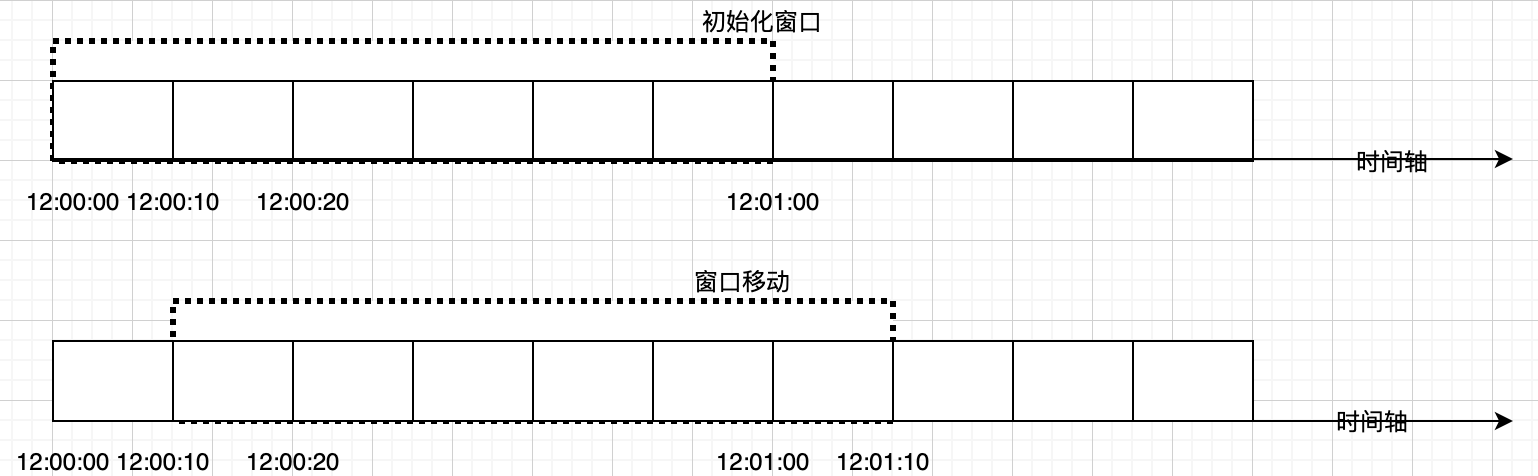#### 原理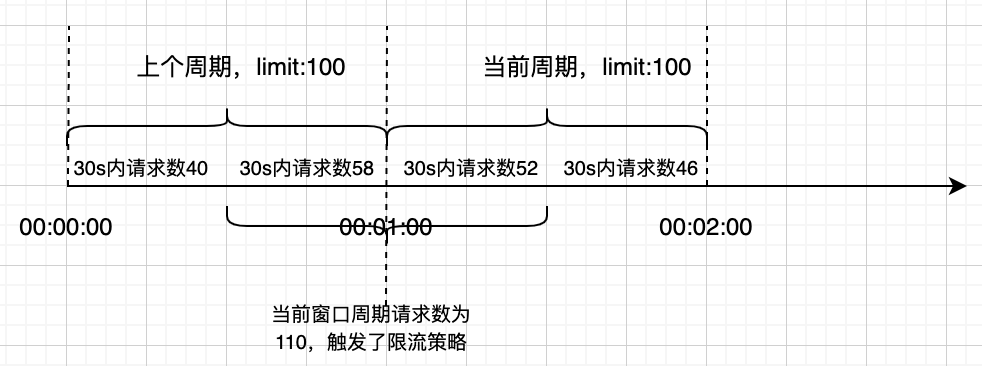#### 实现

class SlidingWindowController { public:  SlidingWindowController(int window_size, int limit, int split_num) {    limit_ = limit;    window_size_ = window_size;    counters_.resize(split_num);    split_num_ = split_num;  }  int IsValid() {    uint64_t now_ms = 0;    GetCurrentTimeMs(&now_ms);    int window_num = std::max(now_ms - window_size_ - start_time_, 0) / (window_size_ / split_num_);    SlidingWindow(window_num);    int count = 0;    for(int i = 0;i < split_num_; ++i){        count += counters_[i];    }    if(count >= limit){      return false;    }else{      counters_[index] ++;      return true;    }    return true;  } private:  void SlidingWindow(int window_num) {    if (window_num == 0) {      return;    }    int slide_num = std::min(window_num, split_num_);    for (int i = 0; i < slide_num; ++i) {      index_ = (index_ + 1) % split_num;      counters_[index_] = 0;    }    start_time_ = start_time_ + wind_num * (window_size_ / split_num_); // 更新滑动窗口时间  }    int window_size_; // 窗口大小，单位为毫秒  int limit_; // 窗口内限流大小  std::vector<int> counters_;  uint64_t start_time_; // 窗口开始时间  int index_ = 0; // 当前窗口计时器索引  int split_num_;};

#### 特点

• 避免了计数器固定窗口算法固定窗口切换时可能会产生两倍于阈值流量请求的问题

• 实现精度依赖于窗口的细分粒度，分的越细，即窗口分块越多，控制的限流越平滑

### 漏桶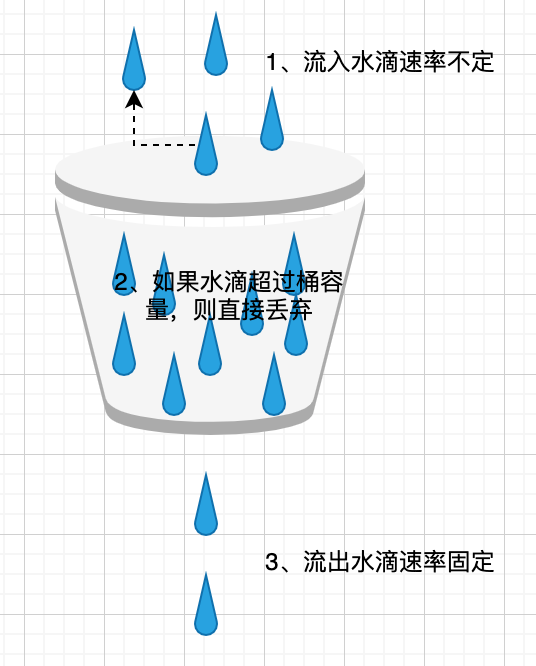#### 实现

class LeakyBucketController { public:  LeakyBucketController(int rate) {    capacity_ = rate;    last_update_time_ = time(nullptr);  }  bool IsValid() {    // 计算这段时间，漏了多少水    uint64_t now = time(nullptr);    int out = (new - last_update_time_) * rate;    if (out > 0) {      last_update_time_ = now;    }    // 计算桶中剩余的水    water_ = std::max(0, water_ - out);    // 如果桶没有满，则表示有效    if (water_ < capacity_) {      ++water_;      return true;    }    return false;  } private:  int capacity_;  int water_ = 0;  uint64_t last_update_time_;};

#### 特点

• 漏桶的漏出速率是固定的由于漏出速率固定，因此即使流量流入速率不定，但是经过漏斗之后，变成了有固定速率的稳定流量，可以对下游系统起到保护作用

• 不能解决流量突发的问题。假设我们设置漏斗速率为 10 个/秒，桶的容量为 50 个。此时突然来了 100 个请求，那么只有 50 个请求被接收，另外 50 个被拒绝。这个时候，你可能会认为瞬间接受了 50 个请求，不就解决了流量突发问题么？不，这 50 个请求只是被接受了，但是没有马上被处理，处理的速度仍然是我们设定的 10 个/秒，所以没有解决流量突发的问题。而接下来我们要谈的令牌桶算法能够在一定程度上解决流量突发的问题。

### 令牌桶

#### 原理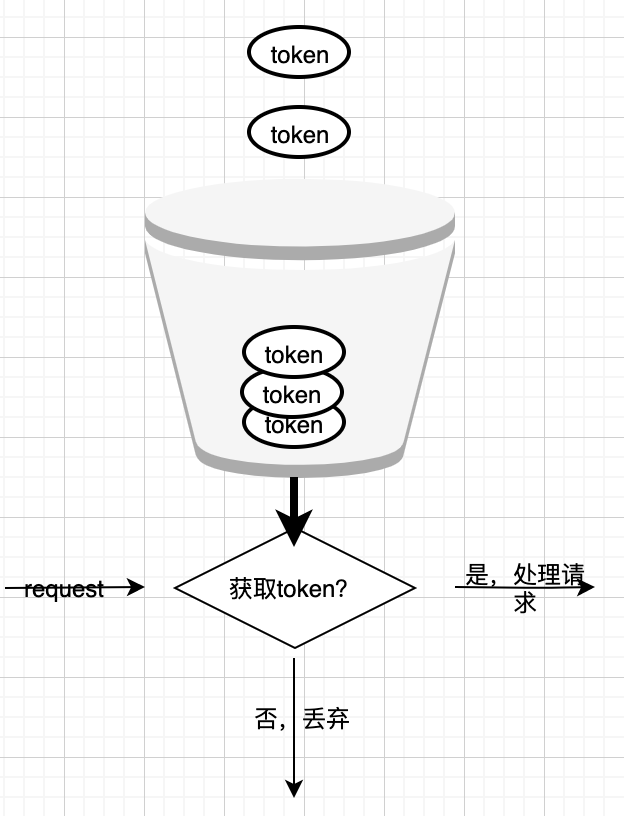#### 实现

class TokenBucketController { public:  TokenBucketController(int num, uint64_t duration) :    duration_(duration), rate_(num > 0 ? (num / duration_ / 1000) : 0),      limit_(num), modulo_(num > 0 ?(num % (duration * 1000)) : 0) {    GetCurrentTimeMs(&last_update_time_);    ::curr_idx = 0;  }  bool IsValid(); private:  void Update();  const uint64_t duration_;  const int rate_;  const int limit_;  const uint64_t modulo_;  uint64_t last_update_time_;  uint64_t loss_ = 0;  uint64_t counts_ = 0;};void TokenBucketController::Update() {  uint64_t cur_time_ms;  GetCurrentTimeMs(&cur_time_ms);  uint64_t time_passed_since_last_update = cur_time_ms - last_update_time_;  if (time_passed_since_last_update == 0) {    return;  }  if (counts_ == static_cast<uint64_t>(limit_)) {    last_update_time_ = cur_time_ms;    return;  }  uint64_t count_to_add = rate_ * time_passed_since_last_update;  loss_ += modulo_ * time_passed_since_last_update;  if (loss_ >= duration_ * 1000) {    count_to_add += loss_ / duration_ / 1000;    loss_ %= (duration_ * 1000);  }  counts_ += count_to_add;  if (counts_ > static_cast<uint64_t>(limit_)) {    counts_ = limit_;  }  last_update_time_ = cur_time_ms;}bool TokenBucketController::IsValid() {  if (limit_ < 0) {    return true;  }  if (counts_ >= 1) {    counts_ -= 1;    return true;  }  Update();  if (counts_ >= 1) {    counts_ -= 1;    return true;  }  return false;}

## 结语## 评论 (1 条评论)15 小时前